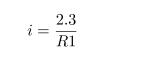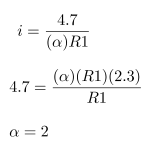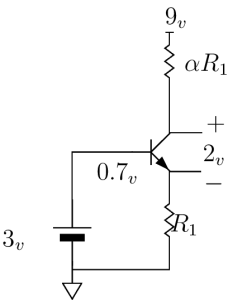# NPN Transistor as an Amplifier :

• We wanted to use the transistor as an amplifier.
• The input voltage at the base was 3v.
• Two resistors, one at the emiiter(R1) and the other at the collector(aR1) were connected.
• 9v supply was given at R1.

Constraints :

• We wanted head room of 2v.
• We wanted to obtain maximum gain.

Design :

• Subtracting 0.7v at the emitter from 3v at the base we get 2.3v.
• Current across R1 is• As we needed 2v head room, the voltage drop across aR1 needed to be 4.7v(ie, 4.7v+2.3v=7v) which is 2v lesser then 9v.
• Current across aR1 is• The maximum gain we can get is a gain of 2.
• Hence the resistor value at the collector should be two times more than the one at the emitter.